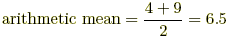index: click on a letter A B C D E F G H I J K L M N O P Q R S T U V W X Y Z A to Z index index: subject areas numbers & symbols sets, logic, proofs geometry algebra trigonometry advanced algebra & pre-calculus calculus advanced topics probability & statistics real world applications multimedia entrieswww.mathwords.com about mathwords website feedback

Arithmetic Mean

The most commonly used type of average. To find the arithmetic mean of a set of n numbers, add the numbers in the set and divide the sum by n.

 Formula:Example: For the numbers 4 and 9,